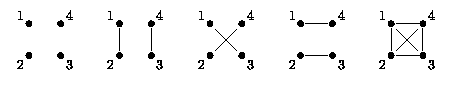시간 제한 메모리 제한 제출 정답 맞은 사람 정답 비율
1 초 128 MB 0 0 0 0.000%

## 문제

An undirected graph with n vertices is called a symmetric labeled cliquer if each connected component of the graph contains the same number of vertices and is a clique, and the vertices of the graph are numbered with numbers from the set {1, ..., n}. Maurycy has drawn all symmetric labeled cliquers on a piece of paper and is going to assess beauty of each of them with a number from the set {1, ..., m} (in particular, different cliquers may be assigned equal grades). In how many ways can he do this? The result should be computed modulo 109 - 401. The figure below depicts all symmetric labeled cliquers for n = 4.## 입력

The only line of the standard input contains two integers n and m (1 ≤ n, m ≤ 2 · 109), separated by a single space and denoting the number of vertices of each symmetric labeled cliquer and the number of grades respectively.

## 출력

The only line of the standard output should contain the number of possible sets of grades modulo 109 - 401.

## 예제 입력 1

4 2


## 예제 출력 1

32